# RD Sharma Solutions for Class 6 Maths Chapter 2: Playing with Numbers Exercise 2.5

## RD Sharma Solutions for Class 6 Maths Exercise 2.5 PDF

We have a set of subject experts who create solutions based on RD Sharma textbook of current CBSE syllabus. The solutions are provided with simple explanations in order to make students understand the concepts. RD Sharma Solutions contain problems solved using various methods to provide better knowledge about the concepts and important formulas. Huge number of examples before each exercise help students analyse the types of problems which would appear in the exam. RD Sharma Solutions for Class 6 Maths Chapter 2 Playing with Numbers Exercise 2.5 are provided here.

## RD Sharma Solutions for Class 6 Maths Chapter 2: Playing with Numbers Exercise 2.5 Download PDF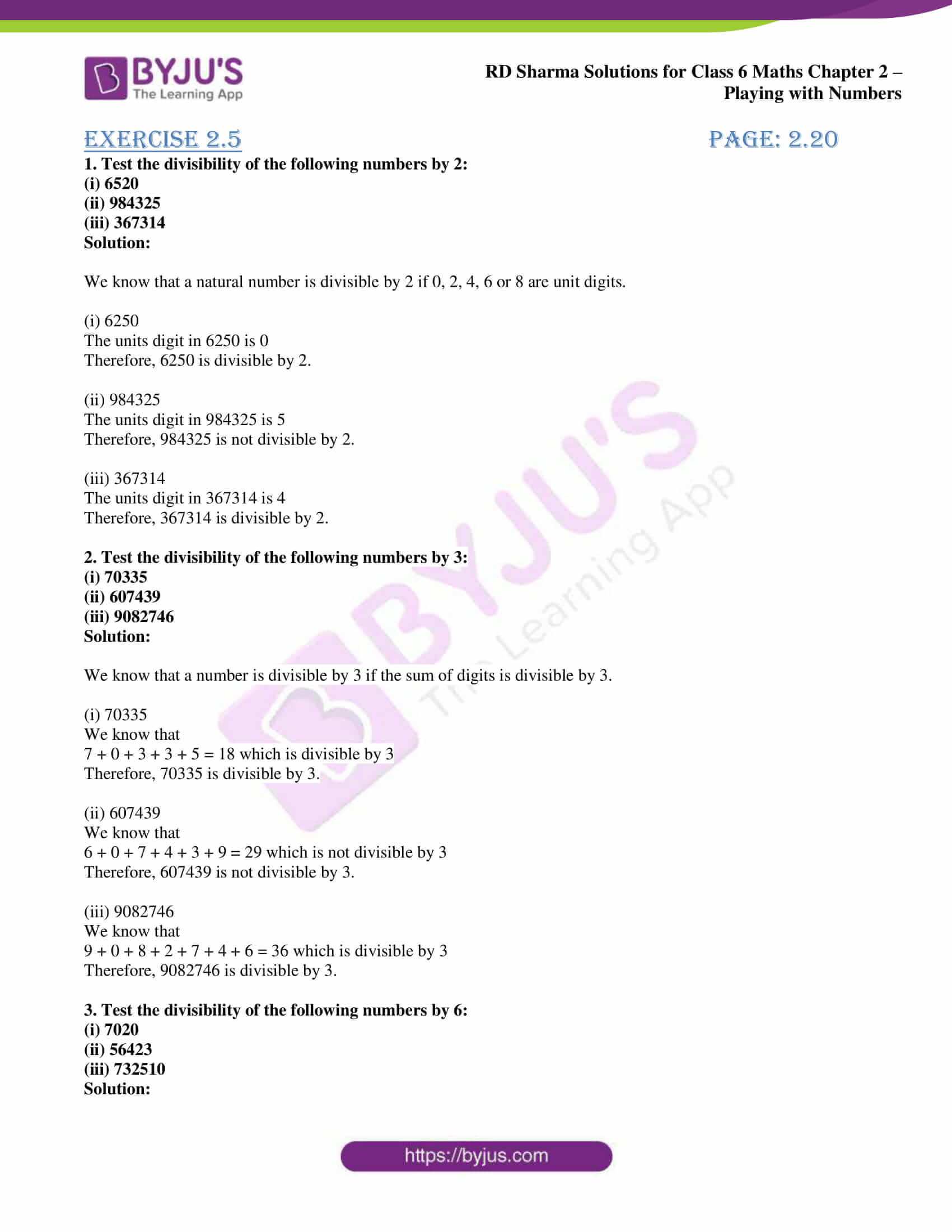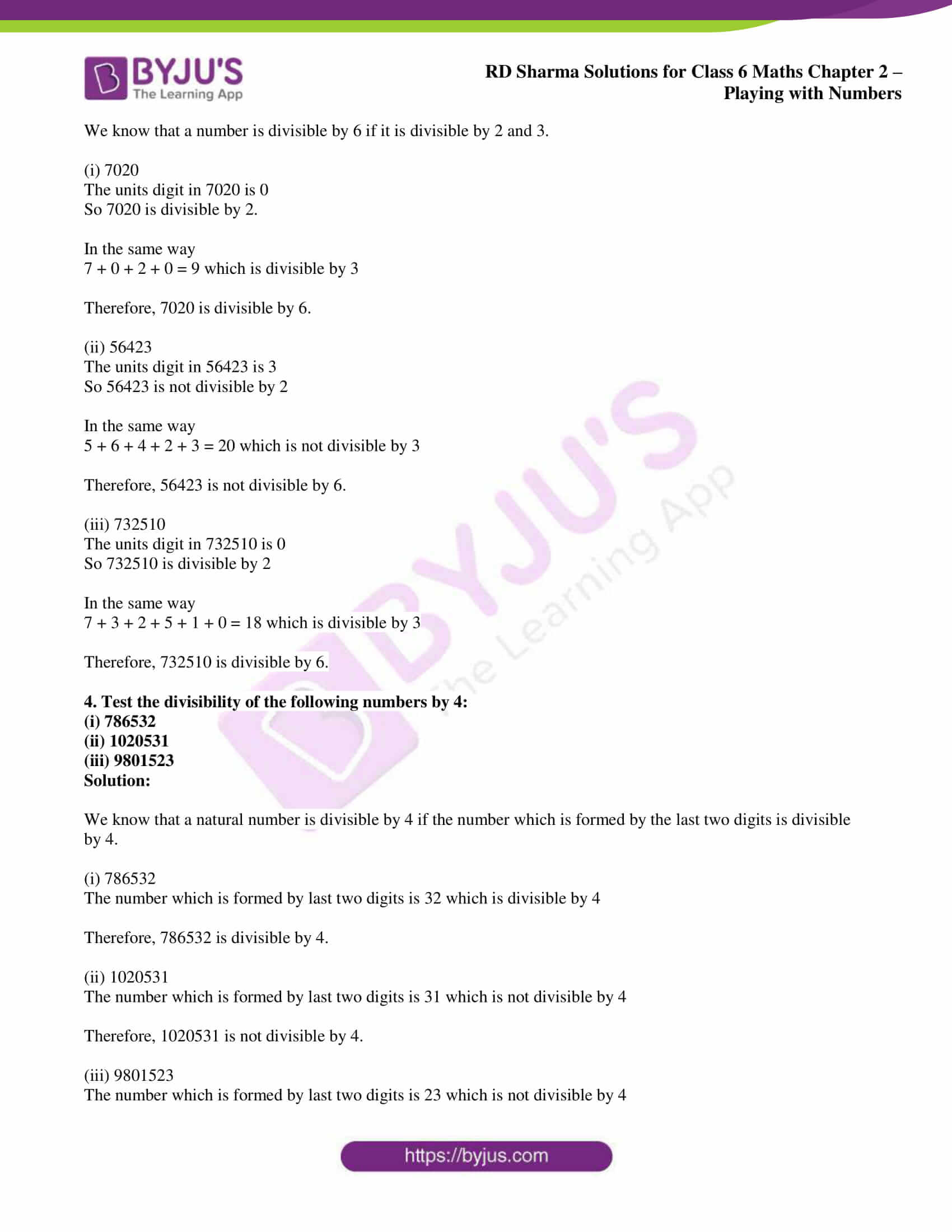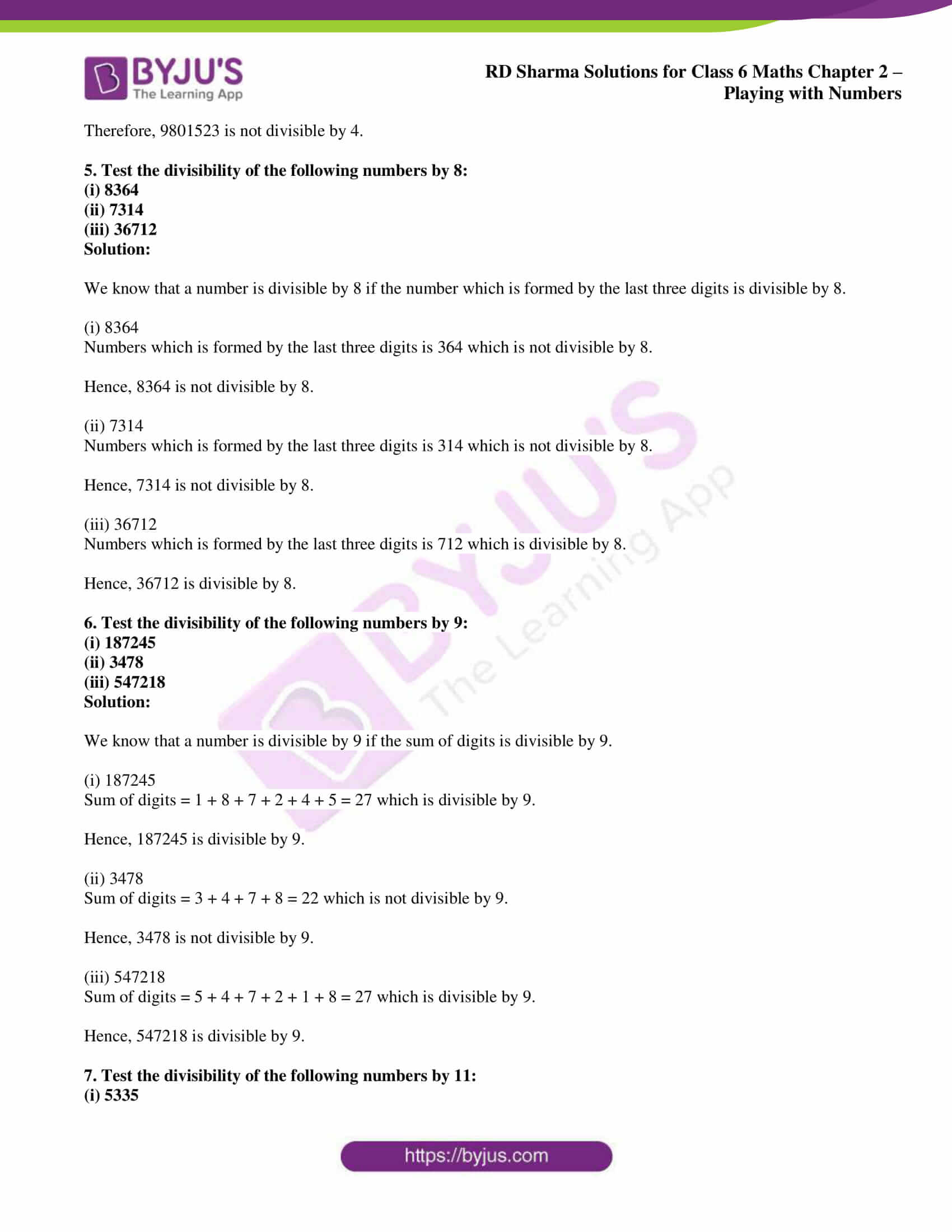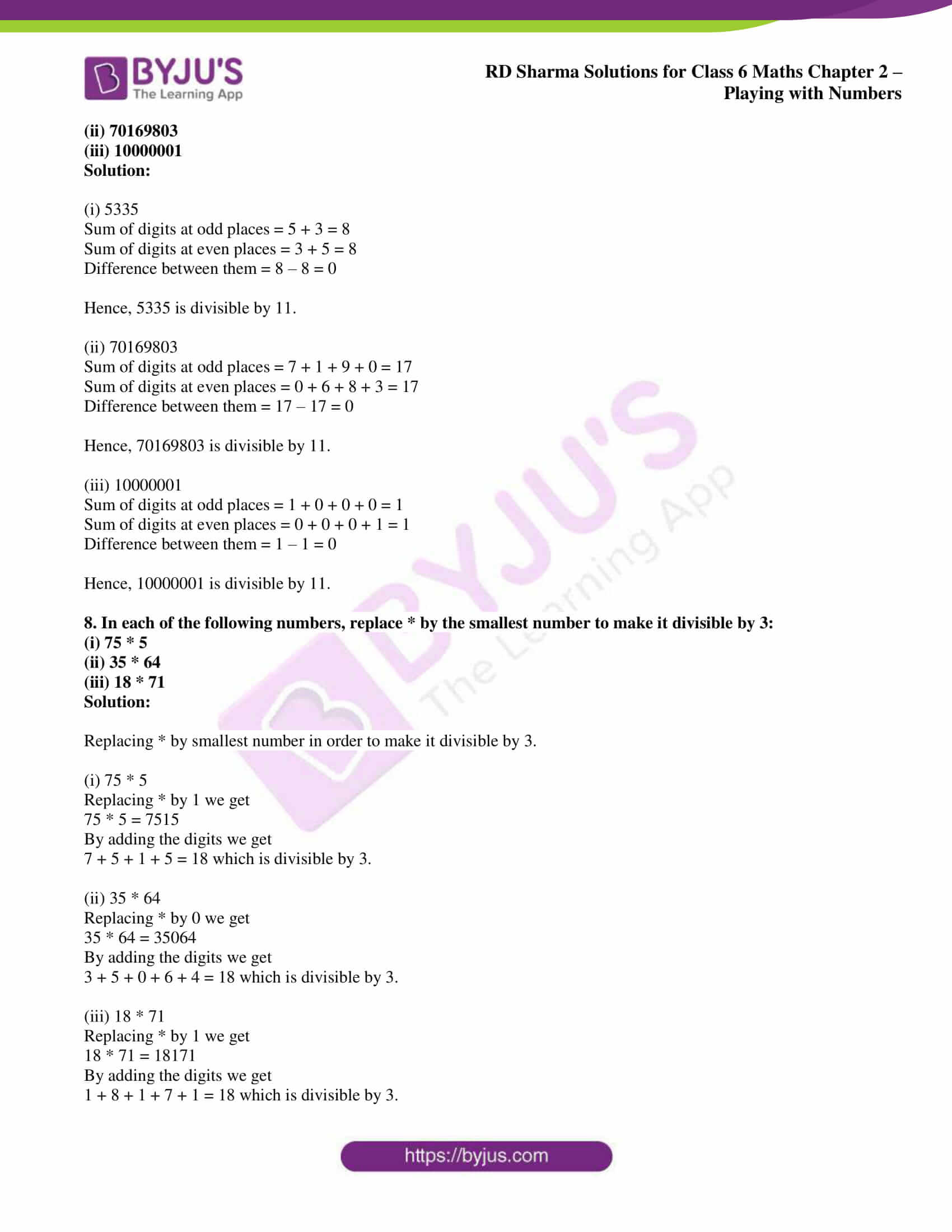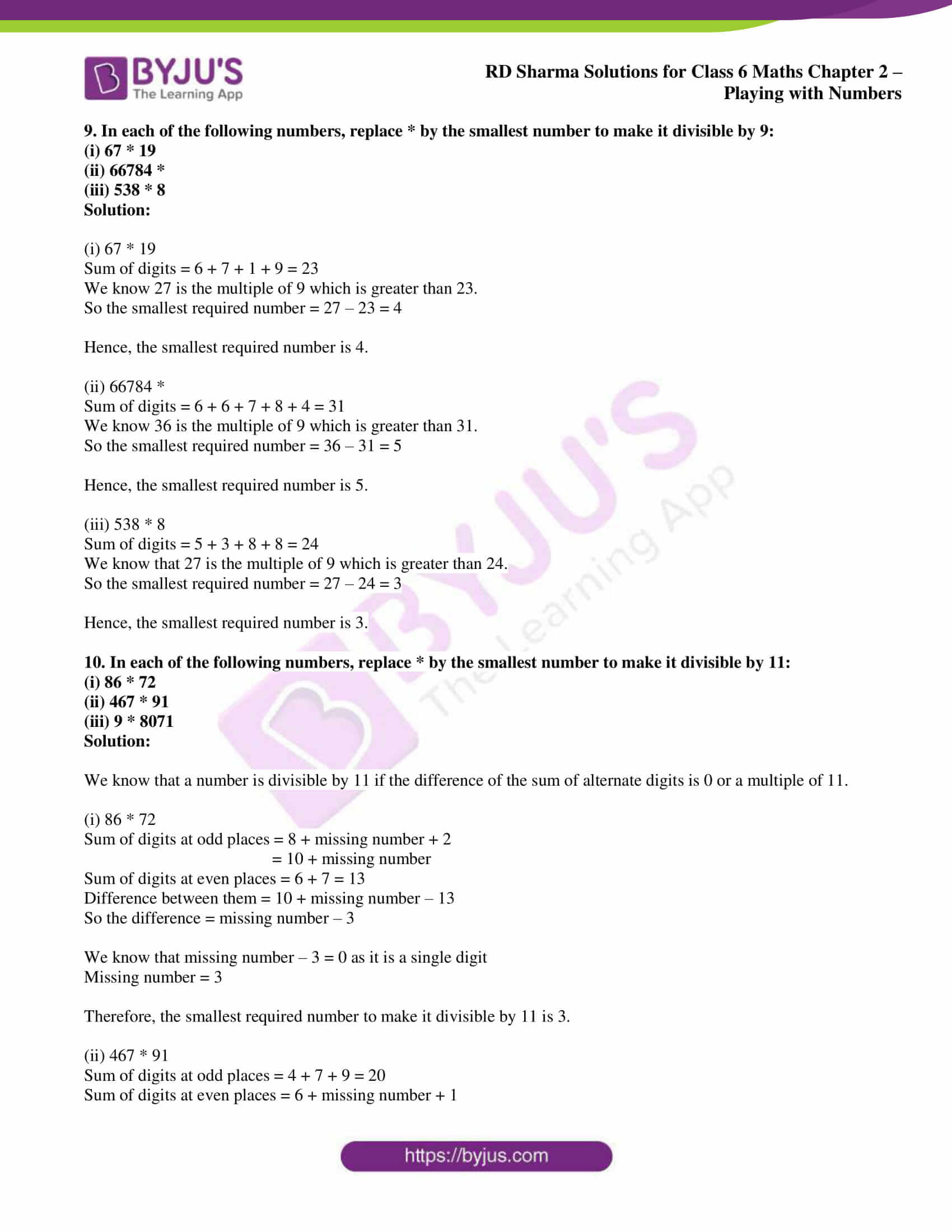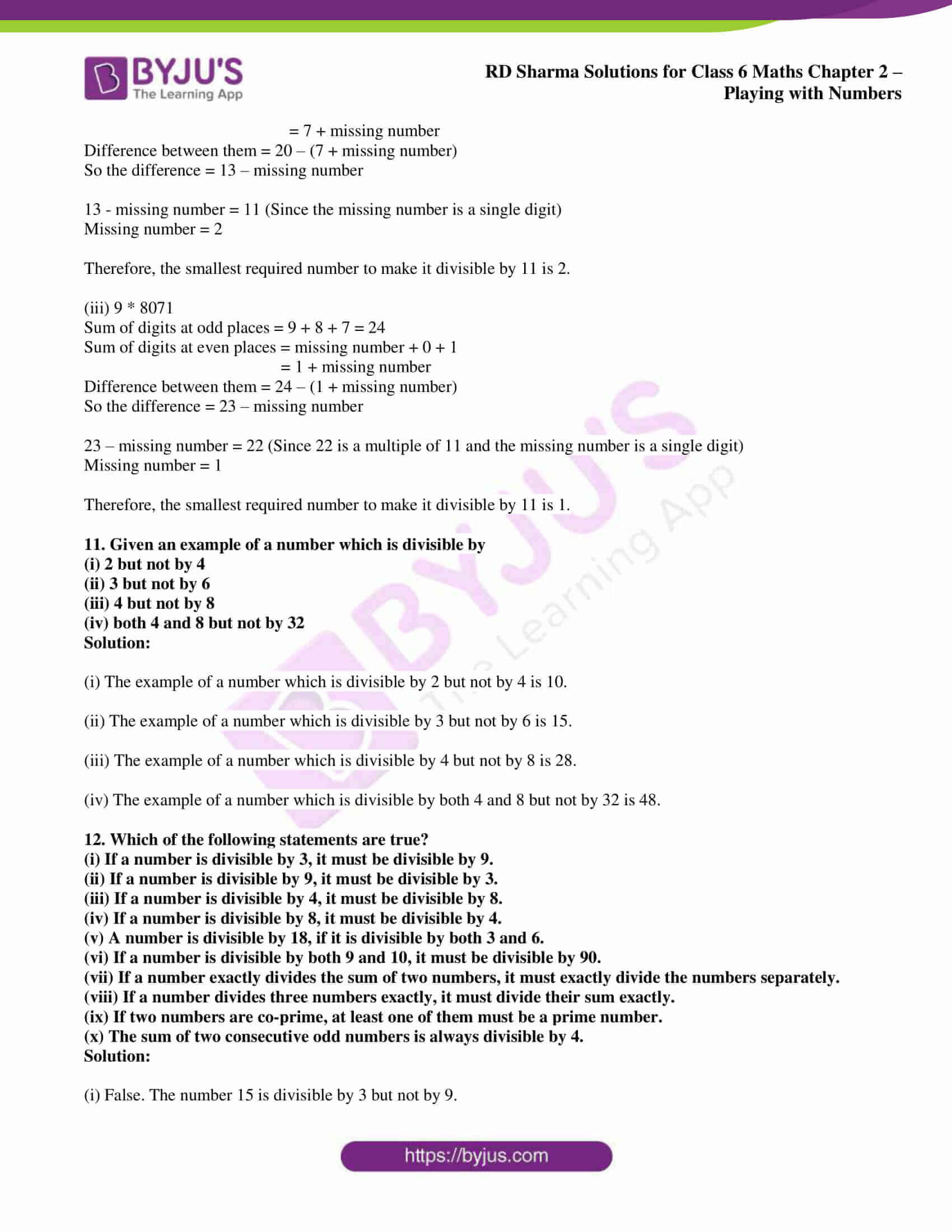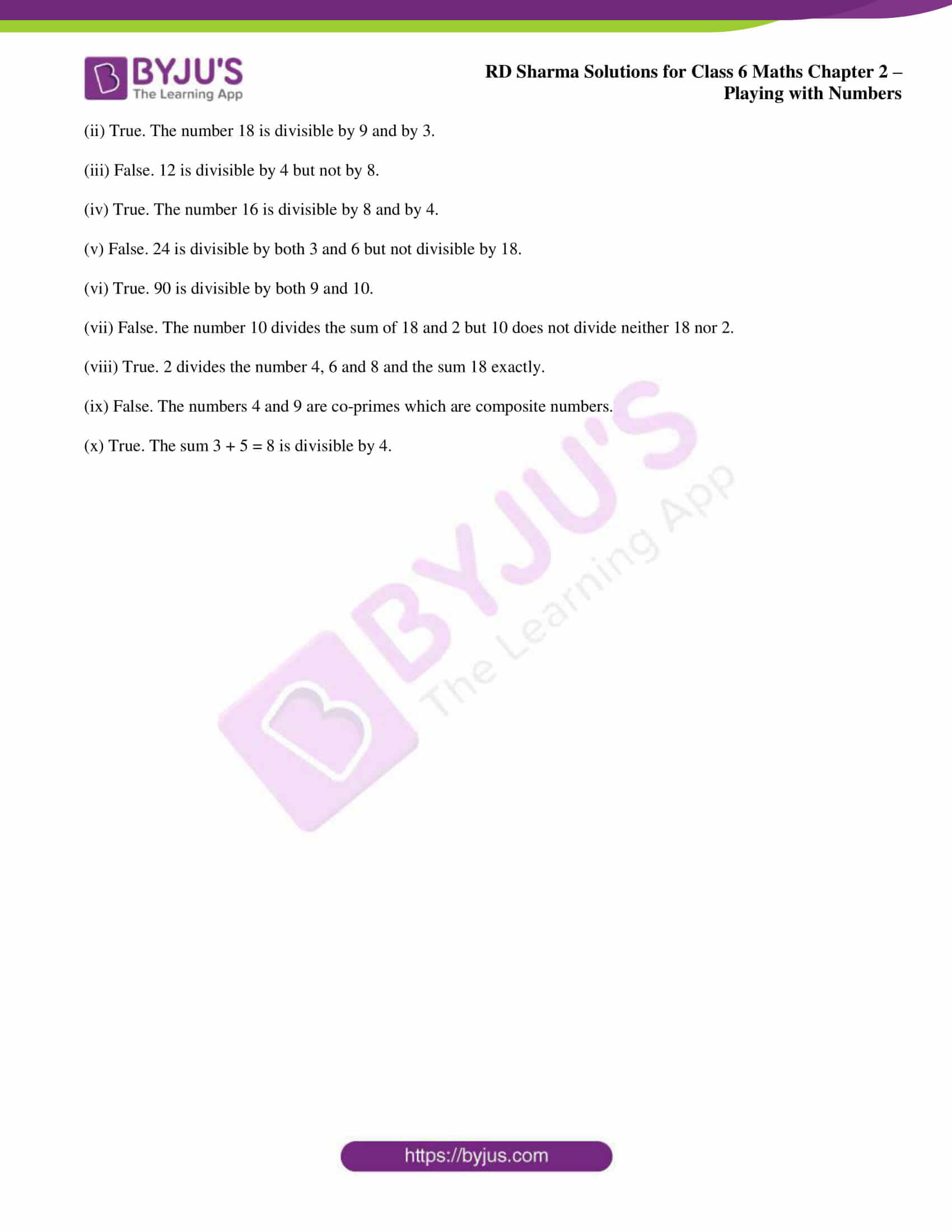## Access answers to Maths RD Sharma Solutions for Class 6 Chapter 2: Playing with Numbers Exercise 2.5

1. Test the divisibility of the following numbers by 2:

(i) 6520

(ii) 984325

(iii) 367314

Solution:

We know that a natural number is divisible by 2 if 0, 2, 4, 6 or 8 are unit digits.

(i) 6250

The units digit in 6250 is 0

Therefore, 6250 is divisible by 2.

(ii) 984325

The units digit in 984325 is 5

Therefore, 984325 is not divisible by 2.

(iii) 367314

The units digit in 367314 is 4

Therefore, 367314 is divisible by 2.

2. Test the divisibility of the following numbers by 3:

(i) 70335

(ii) 607439

(iii) 9082746

Solution:

We know that a number is divisible by 3 if the sum of digits is divisible by 3.

(i) 70335

We know that

7 + 0 + 3 + 3 + 5 = 18 which is divisible by 3

Therefore, 70335 is divisible by 3.

(ii) 607439

We know that

6 + 0 + 7 + 4 + 3 + 9 = 29 which is not divisible by 3

Therefore, 607439 is not divisible by 3.

(iii) 9082746

We know that

9 + 0 + 8 + 2 + 7 + 4 + 6 = 36 which is divisible by 3

Therefore, 9082746 is divisible by 3.

3. Test the divisibility of the following numbers by 6:

(i) 7020

(ii) 56423

(iii) 732510

Solution:

We know that a number is divisible by 6 if it is divisible by 2 and 3.

(i) 7020

The units digit in 7020 is 0

So 7020 is divisible by 2.

In the same way

7 + 0 + 2 + 0 = 9 which is divisible by 3

Therefore, 7020 is divisible by 6.

(ii) 56423

The units digit in 56423 is 3

So 56423 is not divisible by 2

In the same way

5 + 6 + 4 + 2 + 3 = 20 which is not divisible by 3

Therefore, 56423 is not divisible by 6.

(iii) 732510

The units digit in 732510 is 0

So 732510 is divisible by 2

In the same way

7 + 3 + 2 + 5 + 1 + 0 = 18 which is divisible by 3

Therefore, 732510 is divisible by 6.

4. Test the divisibility of the following numbers by 4:

(i) 786532

(ii) 1020531

(iii) 9801523

Solution:

We know that a natural number is divisible by 4 if the number which is formed by the last two digits is divisible by 4.

(i) 786532

The number which is formed by last two digits is 32 which is divisible by 4

Therefore, 786532 is divisible by 4.

(ii) 1020531

The number which is formed by last two digits is 31 which is not divisible by 4

Therefore, 1020531 is not divisible by 4.

(iii) 9801523

The number which is formed by last two digits is 23 which is not divisible by 4

Therefore, 9801523 is not divisible by 4.

5. Test the divisibility of the following numbers by 8:

(i) 8364

(ii) 7314

(iii) 36712

Solution:

We know that a number is divisible by 8 if the number which is formed by the last three digits is divisible by 8.

(i) 8364

Numbers which is formed by the last three digits is 364 which is not divisible by 8.

Hence, 8364 is not divisible by 8.

(ii) 7314

Numbers which is formed by the last three digits is 314 which is not divisible by 8.

Hence, 7314 is not divisible by 8.

(iii) 36712

Numbers which is formed by the last three digits is 712 which is divisible by 8.

Hence, 36712 is divisible by 8.

6. Test the divisibility of the following numbers by 9:

(i) 187245

(ii) 3478

(iii) 547218

Solution:

We know that a number is divisible by 9 if the sum of digits is divisible by 9.

(i) 187245

Sum of digits = 1 + 8 + 7 + 2 + 4 + 5 = 27 which is divisible by 9.

Hence, 187245 is divisible by 9.

(ii) 3478

Sum of digits = 3 + 4 + 7 + 8 = 22 which is not divisible by 9.

Hence, 3478 is not divisible by 9.

(iii) 547218

Sum of digits = 5 + 4 + 7 + 2 + 1 + 8 = 27 which is divisible by 9.

Hence, 547218 is divisible by 9.

7. Test the divisibility of the following numbers by 11:

(i) 5335

(ii) 70169803

(iii) 10000001

Solution:

(i) 5335

Sum of digits at odd places = 5 + 3 = 8

Sum of digits at even places = 3 + 5 = 8

Difference between them = 8 – 8 = 0

Hence, 5335 is divisible by 11.

(ii) 70169803

Sum of digits at odd places = 7 + 1 + 9 + 0 = 17

Sum of digits at even places = 0 + 6 + 8 + 3 = 17

Difference between them = 17 – 17 = 0

Hence, 70169803 is divisible by 11.

(iii) 10000001

Sum of digits at odd places = 1 + 0 + 0 + 0 = 1

Sum of digits at even places = 0 + 0 + 0 + 1 = 1

Difference between them = 1 – 1 = 0

Hence, 10000001 is divisible by 11.

8. In each of the following numbers, replace * by the smallest number to make it divisible by 3:

(i) 75 * 5

(ii) 35 * 64

(iii) 18 * 71

Solution:

Replacing * by smallest number in order to make it divisible by 3.

(i) 75 * 5

Replacing * by 1 we get

75 * 5 = 7515

By adding the digits we get

7 + 5 + 1 + 5 = 18 which is divisible by 3.

(ii) 35 * 64

Replacing * by 0 we get

35 * 64 = 35064

By adding the digits we get

3 + 5 + 0 + 6 + 4 = 18 which is divisible by 3.

(iii) 18 * 71

Replacing * by 1 we get

18 * 71 = 18171

By adding the digits we get

1 + 8 + 1 + 7 + 1 = 18 which is divisible by 3.

9. In each of the following numbers, replace * by the smallest number to make it divisible by 9:

(i) 67 * 19

(ii) 66784 *

(iii) 538 * 8

Solution:

(i) 67 * 19

Sum of digits = 6 + 7 + 1 + 9 = 23

We know 27 is the multiple of 9 which is greater than 23.

So the smallest required number = 27 – 23 = 4

Hence, the smallest required number is 4.

(ii) 66784 *

Sum of digits = 6 + 6 + 7 + 8 + 4 = 31

We know 36 is the multiple of 9 which is greater than 31.

So the smallest required number = 36 – 31 = 5

Hence, the smallest required number is 5.

(iii) 538 * 8

Sum of digits = 5 + 3 + 8 + 8 = 24

We know that 27 is the multiple of 9 which is greater than 24.

So the smallest required number = 27 – 24 = 3

Hence, the smallest required number is 3.

10. In each of the following numbers, replace * by the smallest number to make it divisible by 11:

(i) 86 * 72

(ii) 467 * 91

(iii) 9 * 8071

Solution:

We know that a number is divisible by 11 if the difference of the sum of alternate digits is 0 or a multiple of 11.

(i) 86 * 72

Sum of digits at odd places = 8 + missing number + 2

= 10 + missing number

Sum of digits at even places = 6 + 7 = 13

Difference between them = 10 + missing number – 13

So the difference = missing number – 3

We know that missing number – 3 = 0 as it is a single digit

Missing number = 3

Therefore, the smallest required number to make it divisible by 11 is 3.

(ii) 467 * 91

Sum of digits at odd places = 4 + 7 + 9 = 20

Sum of digits at even places = 6 + missing number + 1

= 7 + missing number

Difference between them = 20 – (7 + missing number)

So the difference = 13 – missing number

13 – missing number = 11 (Since the missing number is a single digit)

Missing number = 2

Therefore, the smallest required number to make it divisible by 11 is 2.

(iii) 9 * 8071

Sum of digits at odd places = 9 + 8 + 7 = 24

Sum of digits at even places = missing number + 0 + 1

= 1 + missing number

Difference between them = 24 – (1 + missing number)

So the difference = 23 – missing number

23 – missing number = 22 (Since 22 is a multiple of 11 and the missing number is a single digit)

Missing number = 1

Therefore, the smallest required number to make it divisible by 11 is 1.

11. Given an example of a number which is divisible by

(i) 2 but not by 4

(ii) 3 but not by 6

(iii) 4 but not by 8

(iv) both 4 and 8 but not by 32

Solution:

(i) The example of a number which is divisible by 2 but not by 4 is 10.

(ii) The example of a number which is divisible by 3 but not by 6 is 15.

(iii) The example of a number which is divisible by 4 but not by 8 is 28.

(iv) The example of a number which is divisible by both 4 and 8 but not by 32 is 48.

12. Which of the following statements are true?

(i) If a number is divisible by 3, it must be divisible by 9.

(ii) If a number is divisible by 9, it must be divisible by 3.

(iii) If a number is divisible by 4, it must be divisible by 8.

(iv) If a number is divisible by 8, it must be divisible by 4.

(v) A number is divisible by 18, if it is divisible by both 3 and 6.

(vi) If a number is divisible by both 9 and 10, it must be divisible by 90.

(vii) If a number exactly divides the sum of two numbers, it must exactly divide the numbers separately.

(viii) If a number divides three numbers exactly, it must divide their sum exactly.

(ix) If two numbers are co-prime, at least one of them must be a prime number.

(x) The sum of two consecutive odd numbers is always divisible by 4.

Solution:

(i) False. The number 15 is divisible by 3 but not by 9.

(ii) True. The number 18 is divisible by 9 and by 3.

(iii) False. 12 is divisible by 4 but not by 8.

(iv) True. The number 16 is divisible by 8 and by 4.

(v) False. 24 is divisible by both 3 and 6 but not divisible by 18.

(vi) True. 90 is divisible by both 9 and 10.

(vii) False. The number 10 divides the sum of 18 and 2 but 10 does not divide neither 18 nor 2.

(viii) True. 2 divides the number 4, 6 and 8 and the sum 18 exactly.

(ix) False. The numbers 4 and 9 are co-primes which are composite numbers.

(x) True. The sum 3 + 5 = 8 is divisible by 4.

### RD Sharma Solutions for Class 6 Maths Chapter 2 – Playing with Numbers Exercise 2.5

RD Sharma Solutions Class 6 Maths Chapter 2 Playing with Numbers Exercise 2.5 has concepts like tests on divisibility of numbers and their general properties.

### Key features of RD Sharma Solutions for Class 6 Maths Chapter 2: Playing with Numbers Exercise 2.5

• Using the PDF of solutions, the students can grasp the important formulas which are highlighted in a shorter period of time.
• Solving the problems and illustrative examples help students understand the types of questions that would arise in the exam.
• By using PDF as a reference guide, the students can solve the exercise wise problems according to the CBSE syllabus.
• The exercise wise questions help students understand the topics which are important from the exam perspective.